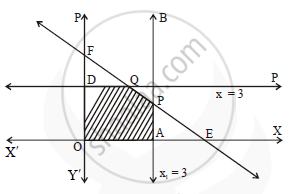# Solve the following L.P.P graphically: Maximize :Z = 10x + 25y Subject to : x ≤ 3, y ≤ 3, x + y ≤ 5, x ≥ 0, y ≥ 0 - Mathematics and Statistics

Graph
Sum

Solve the following L.P.P graphically:

Maximize: Z = 10x + 25y
Subject to: x ≤ 3, y ≤ 3, x + y ≤ 5, x ≥ 0, y ≥ 0

#### Solution

First we draw the lines AB, CD and EF whose equations are x = 3, y = 3 and x + y = 5 respectively.

 Line Equation Point on the X-axis Point on the Y-axis Sign Region AB x = 3 A(3,0) - ≤ origin side of line AB CD y = 3 - D(0,3) ≤ origin side of line CD EF x + y = 5 E(5,0) F(0,5) ≤ origin side of line EFThe feasible region is OAPQDO which is shaded in the figure.
The vertices of the feasible region are O (0,0), A (3, 0), P, Q and D (0, 3)
P is the point of intersection of the lines x + y = 5 and x = 3
Substituting x = 3 in x + y = 5, we get,

3+ y=5

y = 2
P≡ (3, 2)
Q is the point of intersection of the lines x + y = 5 and y = 3
Substituting y = 3 in x + y = 5, we get,
x + 3 = 5

x = 2
Q ≡ (2,3)
The values of the objective function z = 10x + 25y at these vertices are

Z(O)  =10(0)+ 25(0)= 0
Z(A)  =10(3) + 25(0) = 30
Z(P)  =10(3) + 25(2) = 30 + 50 = 80
Z(Q)  =10(2) + 25(3) = 20 + 75 = 95
Z(D) =10(0) + 25(3) =75

Z has max imumvalue 95, when x = 2 and y = 3.

Concept: Graphical Method of Solving Linear Programming Problems
Is there an error in this question or solution?
Chapter 7: Linear Programming - Exercise 7.4 [Page 241]

Share